# 31 Parts of Lists

 31 Parts of Lists
Part lets you pick out an element of a list.
Pick out element 2 from a list:
Part[{a, b, c, d, e, f, g}, 2]

[[...]] is an alternative notation.
Use [] to pick out element 2 from a list:
{a, b, c, d, e, f, g}[]

Negative part numbers count from the end of a list:
{a, b, c, d, e, f, g}[[-2]]

You can ask for a list of parts by giving a list of part numbers.
Pick out parts 2, 4 and 5:
{a, b, c, d, e, f, g}[[{2, 4, 5}]]

;; lets you ask for a span or sequence of parts.
Pick out parts 2 through 5:
{a, b, c, d, e, f, g}[[2 ;; 5]]

Take the first 4 elements from a list:
Take[{a, b, c, d, e, f, g}, 4]

Take the last 2 elements from a list:
Take[{a, b, c, d, e, f, g}, -2]

Drop[{a, b, c, d, e, f, g}, -2]

Let’s now talk about lists of lists, or arrays. Each sublist acts as a row in the array.
{{a, b, c}, {d, e, f}, {g, h, i}} // Grid

Pick out the second sublist, corresponding to the second row, in the array:
{{a, b, c}, {d, e, f}, {g, h, i}}[]

This picks out element 1 on row 2:
{{a, b, c}, {d, e, f}, {g, h, i}}[[2, 1]]

It’s also often useful to pick out columns in an array.
Pick out the first column, by getting element 1 on all rows:
{{a, b, c}, {d, e, f}, {g, h, i}}[[All, 1]]

The function Position finds the list of positions at which something appears.
Here there’s only one d, and it appears at position 2, 1:
Position[{{a, b, c}, {d, e, f}, {g, h, i}}, d]

This gives a list of all positions at which x appears:
Position[{{x, y, x}, {y, y, x}, {x, y, y}, {x, x, y}}, x]

The positions at which “a” occurs in a list of characters:
Position[Characters["The Wolfram Language"], "a"]

Flatten[Position[IntegerDigits[2^500], 0]]

The function ReplacePart lets you replace parts of a list:
Replace part 3 with x:
ReplacePart[{a, b, c, d, e, f, g}, 3 -> x]

Replace two parts:
ReplacePart[{a, b, c, d, e, f, g}, {3 -> x, 5 -> y}]

Replace 5 randomly chosen parts with “--”:
ReplacePart[Characters["The Wolfram Language"], Table[RandomInteger[{1, 20}] -> "--", 5]]

Sometimes one wants particular parts of a list to just disappear. One can do this by replacing them with Nothing.
Nothing just disappears:
{1, 2, Nothing, 4, 5, Nothing}

Replace parts 1 and 3 with Nothing:
ReplacePart[{a, b, c, d, e, f, g}, {1 -> Nothing, 3 -> Nothing}]

Take 50 random words, dropping ones longer than 5 characters, and reversing others:
If[StringLength[#] > 5, Nothing, StringReverse[#]] & /@ RandomSample[WordList[], 50]

Take takes a specified number of elements in a list based on their position. TakeLargest and TakeSmallest take elements based on their size.
Take the 5 largest elements from a list:
TakeLargest[Range, 5]

TakeLargestBy and TakeSmallestBy take elements based on applying a function.
From the first 100 Roman numerals take the 5 that have the largest string length:
TakeLargestBy[Array[RomanNumeral, 100], StringLength, 5]

 Part[list,n] part n of a list list[[n]] short notation for part n of a list list[[{n1,n2,...}]] list of parts n1, n2, ... list[[n1;;n2]] span (sequence) of parts n1 through n2 list[[m,n]] element from row m, column n of an array list[[All,n]] all elements in column n Take[list,n] take the first n elements of a list TakeLargest[list,n] take the largest n elements of a list TakeSmallest[list,n] take the smallest n elements of a list TakeLargestBy[list,f,n] take elements largest by applying f TakeSmallestBy[list,f,n] take elements smallest by applying f Position[list,x] all positions of x in list ReplacePart[list,nx] replace part n of list with x Nothing a list element that is automatically removed
31.1Find the last 5 digits in 2^1000. »
Expected output:
 Out[]=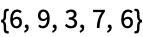Answer & check your solution
31.2Pick out letters 10 through 20 in the alphabet. »
Expected output:
 Out[]=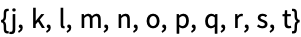Answer & check your solution
31.3Make a list of the letters at even-numbered positions in the alphabet. »
Expected output:
 Out[]=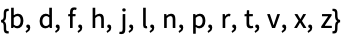Answer & check your solution
31.4Make a line plot of the second to last digit in the first 100 powers of 12. »
Expected output:
 Out[]=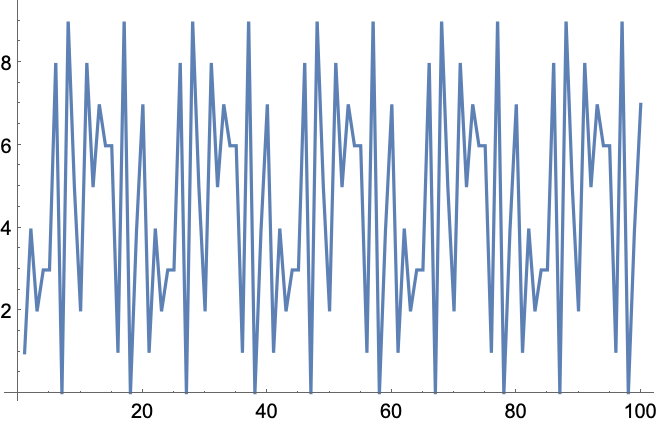Answer & check your solution
31.5Join lists of the first 20 squares and cubes, and get the 10 smallest elements of the combined list. »
Expected output:
 Out[]=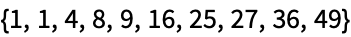Answer & check your solution
31.6Find the positions of the word “software” in the Wikipedia entry for “computers”. »
Sample expected output:
 Out[]=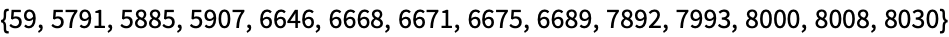Answer & check your solution
Sample expected output:
 Out[]=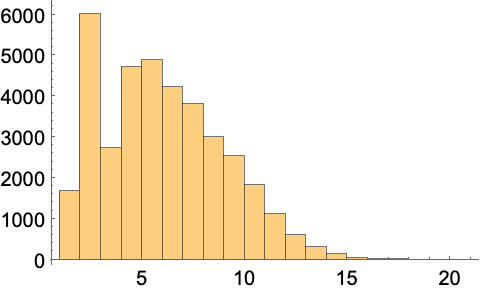Answer & check your solution
Expected output:
 Out[]=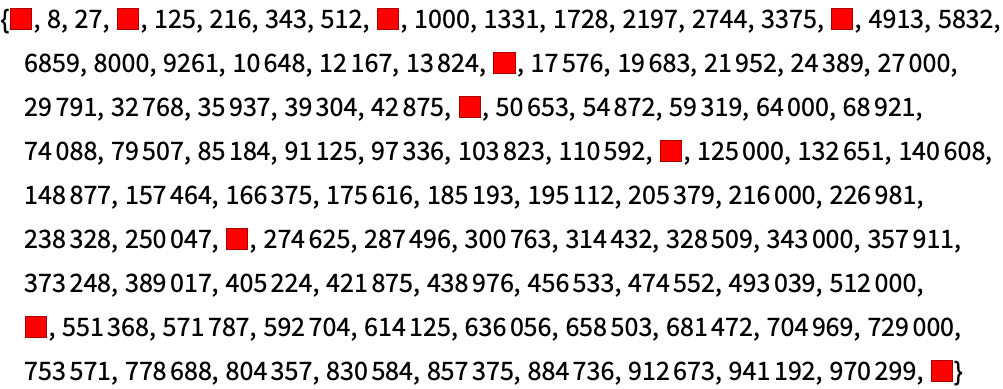Answer & check your solution
31.9Make a list of the first 100 primes, dropping ones whose first digit is less than 5. »
Expected output:
 Out[]=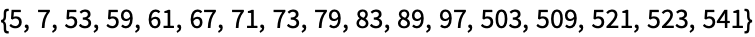Answer & check your solution
31.10Make a grid starting with Range, then at each of 9 steps randomly removing another element. »
Sample expected output:
 Out[]=Answer & check your solution
31.11Find the longest 10 words in WordList[ ]»
Sample expected output:
 Out[]=Answer & check your solution
31.12Find the 5 longest integer names for integers up to 100. »
Expected output:
 Out[]=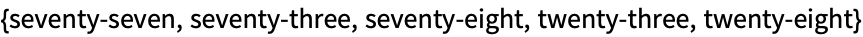Answer & check your solution
31.13Find the 5 English names for integers up to 100 that have the largest number of “e”s in them. »
Expected output:
 Out[]=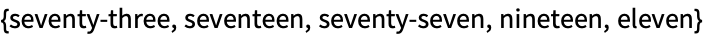Answer & check your solution
How does one read list[[n]] out loud?
Usually “list part n” or “list sub n”. The second form (with “sub” short for “subscript”) comes from thinking about math and extracting components of vectors.
What happens if one asks for a part of a list that doesn’t exist?
One gets a message, and the original computation is returned undone.
Can I just get the first position at which something appears in a list?
How can I insert a sequence of elements into a list?
A convenient way is to replace one element with Splice[{x, y, ...}], which automatically “splices itself” in, so that for example {a, b, Splice[{x, y}], c} gives {a, b, x, y, c}.
• First and Last are equivalent to [] and [[-1]].
• In specifying parts, 1;;-1 is equivalent to All.